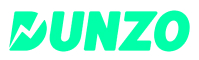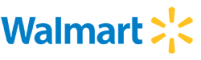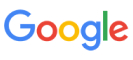New update is available. Click here to update.
Topics

# Word Break-1

Hard0/120
Average time to solve is 36m+15 more companies

## Problem statement

You are given a string 's', and a dictionary of words 'dict' containing 'n' words. Your task is to add spaces in 's' to form valid sentences, where each word is a word from the dictionary.

You need to return all possible sentences that can be formed using the given dictionary.

Note :
``````The same word from a dictionary can be used as many times as possible to make sentences.
``````
Detailed explanation ( Input/output format, Notes, Images )
Sample Input 1:
``````6
god
is
now
no
where
here
godisnowherenowhere
``````
Sample Output 1:
``````god is no where no where
god is no where now here
god is now here no where
god is now here now here
``````
Explanation to Sample Input 1:
``````One way to make sentences is to take “god” and append a space, then take “is”  and append space, take “now” from the dictionary and take “here” as well.
Similarly, for other sentences, also we can add space to get other possible sentences.
Note that you can reuse dictionary words as “no” and “now” are used two times to make the same sentence.
``````
Sample Input 2:
``````4
god
is
no
here
godisnowhere
``````
Sample Output 2:
``````-1
``````
Explanation to Sample Input 2::
``````It is not possible to break the string 's' in a way that all the words are present in the dictionary 'dict' therefore output is -1.
``````
Constraints :
``````1 <= n <= 1000
1 <= dic[i] <= 10
1 <= s.length <= 20

Time limit: 1 sec.
``````Console